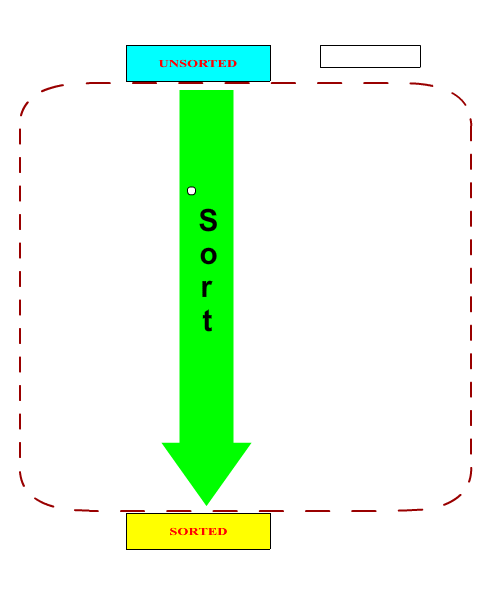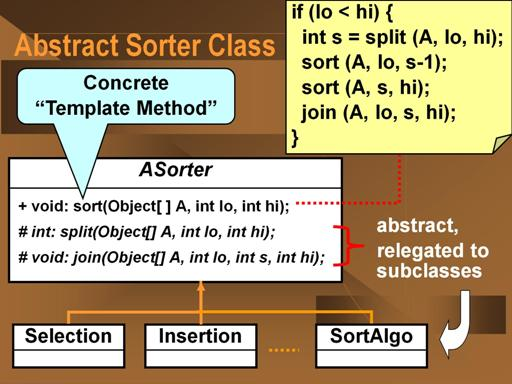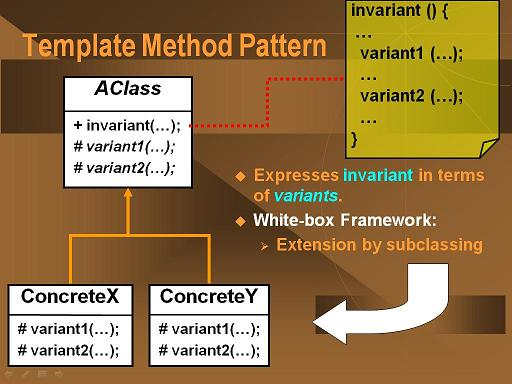# Design Patterns for Sorting

(Back to Connexions Modules Home)

Summary: In 1985, Susan Merritt proposed a new taxonomy for comparison-based sorting algorithms. At the heart of Merritt's thesis is the principle of divide and conquer. Merritt's thesis is potentially a very powerful method for studying and understanding sorting. However, the paper did not offer any concrete implementation of the proposed taxonomy. The following is our object-oriented formulation and implementation of Merritt's taxonomy.

The following discussion is based on the the SIGCSE 2001 paper by Nguyen and Wong, "Design Patterns for Sorting"1.

## Merritt's Thesis

In 1985, Susan Merritt proposed that all comparison-based sorting could be viewed as “Divide and Conquer” algorithms.2 That is, sorting could be thought of as a process wherein one first "divides" the unsorted pile of whatever needs to sorted into smaller piles and then "conquers" them by sorting those smaller piles. Finally, one has to take the the smaller, now sorted piles and recombines them into a single, now-sorted pile.

We thus end up with a recursive definition of sorting:

• To sort a pile:
• Split the pile into smaller piles
• Sort the smaller piles <object type="application/x-shockwave-flash" data="split-join.swf" height="625" width="750"><param name="movie" value="split-join.swf"/><embed src="split-join.swf" type="application/x-shockwave-flash" pluginspace="http://www.macromedia.com/shockwave/download/index.cgi?P1_Prod_Version=ShockwaveFlash" height="625" width="750"/></object>
• Join the sorted smaller piles into a single pile

We can see Merritt's recursive notion of sorting as a split-sort-join process in a pictoral manner by considering the general sorting process as a "black box" process that takes an unsorted set and returns a sorted set. Merritt's thesis thus contends that this sorting process can be described as a splitting followed by a sorting of the smaller pieces followed by a joining of the sorted pieces. The smaller sorting process can thus be similarly described. The base case of this recursive process is when the set has been reduced to a single element, upon which the sorting process cannot be broken down any more as it is a trivial no-op.

Figure 1: Sorting can be seen as a recursive process that splits the unsorted items into multiple unsorted sets, sorts them and then rejoins the now sorted sets. When a set is reduced to a single element (blank boxes above), sorting is a trivial no-op.
Animation of the Merritt Sorting Thesis (Click the "Reveal More" button)Merritt's thesis is potentially a very powerful method for studying and understanding sorting. In addition, Merritt's abstract characterization of sorting exhibits much object-oriented (OO) flavor and can be described in terms of OO concepts.

## Capturing the Abstraction

So, how do we capture the abstraction of sorting as described by Merritt? Fundamentally, we have to recognize that the above description of sorting contains two distinct parts: the invariant process of splitting into sub-piles, sorting the sub-piles and joining the sub-piles, and the variant processes of the actual splitting and joining algorithms used.

Here, we will restrict ourselves to the process of sorting an array of objects, in-place -- that is, the original array is mutated from unsorted to sorted (as opposed to returning a new array of sorted values and leaving the original untouched). The `Comparator` object used to compare objects will be given to the sorter's constructor.

Figure 2: The invariant sorting process is represented as an abstract class
Abstract Sorter ClassHere, the invariant process is represented by the concrete `sort` method, which performs the split-sort-sort-join process as described by Merritt. The variant processes are represented by the abstract `split` and `join` methods, whose exact behaviors are indeterminate at this time.

Above the methods are defined as following:

`final void sort(Object [] A, int lo, int hi)` -- sorts the given unsorted array of objects, `A`, defined from index `lo` to index `hi`, inclusive. This method is implemented here and marked `final` to enforce its invariance with respect to the subclasses. It is this method that implements Merritt's split-sort-join process.

`abstract int split(Object [] A, int lo, int hi)` -- splits the given unsorted array of objects, `A`, defined from index `lo` to index `hi`, inclusive, into two adjacent sub-arrays. The returned index is the index of the first element of the upper sub-array. The implementation of this abstract method is in the sub-classes.

`abstract void join(Object [] A, int lo, int s, int hi)` -- joins two sorted adjacent sub-arrays of objects in the array `A`, where the lower sub-array is from index `lo` to index `s`, inclusive, and the upper sub-array is from index `s` to index `hi`, inclusive. The implementation of this abstract method is in the subclasses.

Here's the full code for the abstract `ASorter` class, the abstract superclass for all concrete sorters and the implementation of Merritt's template for sorting:

## ASorter class

```package sorter;

public abstract class ASorter
{
protected AOrder aOrder;
/**
* The constructor for this class.
* @param aOrder The abstract ordering strategy to be used by any subclass.
*/
protected ASorter(AOrder aOrder)
{
this.aOrder = aOrder;
}

/**
* Sorts by doing a split-sort-sort-join.  Splits the original array into two subarrays,
* recursively sorts the split subarrays, then re-joins the sorted subarrays together.
* This is the template method.  It calls the abstract methods split and join to do
* the work.  All comparison-based sorting algorithms are concrete subclasses with
* specific split and join methods.
* @param A the array A[lo:hi] to be sorted.
* @param lo the low index of A.
* @param hi the high index of A.
*/
public final void sort(Object[] A, int lo, int hi)
{
if (lo < hi)
{
int s = split (A, lo, hi);
sort (A, lo, s-1);
sort (A, s, hi);
join (A, lo, s, hi);
}
}

/**
* Splits A[lo:hi] into A[lo:s-1] and A[s:hi] where s is the returned value of this function.
* @param A the array A[lo:hi] to be sorted.
* @param lo the low index of A.
* @param hi the high index of A.
*/
protected abstract int split(Object[] A, int lo, int hi);

/**
* Joins sorted A[lo:s-1] and sorted A[s:hi] into A[lo:hi].
* @param A A[lo:s-1] and A[s:hi] are sorted.
* @param lo the low index of A.
* @param hi the high index of A.
*/
protected abstract void join(Object[] A, int lo, int s, int hi);

/**
* An accessor method for the abstract ordering strategy.
* @param aOrder
*/
public void setOrder(AOrder aOrder)
{
this.aOrder = aOrder;
}
}
```
Note: `AOrder` is an abstract ordering operator whose concrete implementations define the binary ordering for the object being sorted. The examples below, only use the `AOrder.lt(Object x, Object y)` method, which returns `true` if `x < y`. The sorting framework could easily be modified to use `java.util.Comparator` instead with no loss of generality.

## Template Design Pattern

The invariant sorting process as described by Merritt is an example of the Template Method Design Pattern.

Figure 3: The Template Method Design Pattern describes an invariant concrete process in terms of variant, abstract methods.
Template Method Design PatternHere, the invariant process is represented by a concrete method of an abstract superclass. This concrete method's implementation is in terms of abstract methods of the same class. These abstract methods represent the variant processes and are implemented in the sub-classes. This type of class organization where the variant processes are relegated to sub-classes is also known as a white box framework.

## Concrete Sorters

In order to create a sorter that can actually perform a sorting operation, we need to subclass the above `ASorter` class and implement the abstract `split` and `join` methods. It should be noted that in general, the `split` and `join` methods form a matched pair. One can argue that it is possible to write a universal join methods (a merge operation) but it would be highly inefficent in most cases.

### Example 1: Selection Sort

Tradionally, an in-place selection sort is performed by selecting the smallest (or largest) value in the array and placing it in the right-most location by either swapping it with the right-most element or by shifting all the in-between elements to the left. The selection and swapping/shifting process then repeated with the sub-array to the left of the newly placed element. This continues until only one element remains in the array. A selection sort is commonly used to do something like a sort group of people into ascending height.

Below is an animation of a traditional selection sort algorithm:

Figure 4: The extrema values are removed from an ever-shrinking unordered set and placed into the resulting sorted array. Here, the smallest values are removed from the left and placed to the right in the array.In terms of the Merritt sorting paradigm, a selection sort can be broken down into a splitting process that is the same as the above selection process and a trivial join process. Looking at the above selection and swap/shift process, we see that it is describing a the splitting off of a single element, the smallest, from an array. The process repeats recursively until there is nothing more to split off. The sorting of a single element is a no-op, so after that the recursion rolls back out though the joining process. But the joining process is trivial, a no-op, because the elements are already in their corret positions. The beauty of Merritt's insight is the realize that by considering a no-op as an operational part of a process, all the different types of binary comparison-based sorting could be unified under a common framework.

Below is an animation of a Merritt selection sort algorithm:

Figure 5: The splitting process splits off one element at a time, the smallest element, from the left and placed to the right in the array. The join process is a no-op because the elements are already in their correct places.
Merritt Selection Sort ProcessThe code to implement a selection sorter is straightforward. One need only implement the `split` and `join` methods where the split method always returns the `lo+1` index because the smallest value in the (sub-)array has been moved to the index `lo` position. Because the bulk of the work is being done in the splitting method, selection sort is classified as an "hard split, easy join" sorting process. In the Java implementation of the SelectionSorter class below, the `split` method splits off the extrema (minimum, here) value from the sub-array, while the `join` method is a no-op.

#### SelectionSorter class

```package sorter;

/**
* A concrete sorter that uses the Selection Sort technique.
*/
public class SelectionSorter extends ASorter
{

/**
* The constructor for this class.
* @param iCompareOp The comparison strategy to use in the sorting.
*/
public SelectionSorter(AOrder iCompareOp)
{
super(iCompareOp);
}
/**
* Splits A[lo:hi] into A[lo:s-1] and A[s:hi] where s is the returned value of this function.
* This method places the "smallest" value in the lo position and splits it off.
* @param A the array A[lo:hi] to be sorted.
* @param lo the low index of A.
* @param hi the high index of A.
* @return lo+1 always
*/
protected int split(Object[] A, int lo, int hi)
{
int s = lo;
int i = lo + 1;
// Invariant: A[s] <= A[lo:i-1].
// Scan A to find minimum:
while (i <= hi)
{
if (aOrder.lt(A[i], A[s]))
s = i;
i++; // Invariant is maintained.
} // On loop exit: i = hi + 1; also invariant still holds;
// this makes A[s] the minimum of A[lo:hi].

// Swapping A[lo] with A[s]:
Object temp = A[lo];
A[lo] = A[s];
A[s] = temp;
return lo + 1;
}

/**
* Joins sorted A[lo:s-1] and sorted A[s:hi] into A[lo:hi].
* This method does nothing.  The sub-arrays are already in proper order.
* @param A A[lo:s-1] and A[s:hi] are sorted.
* @param lo the low index of A.
* @param s
* @param hi the high index of A.
*/
protected void join(Object[] A, int lo, int s, int hi)
{
}
}
```

What's interesting to note here is what is missing from the above code. A tradional selection sort aalgorithm is implemented using a nested double loop, one to find the smallest value and one to repeatedly process the ever-shrinking unsorted sub-array. Notice that the above code only has a single loop, which coresponds to the inner loop of a traditional implementation. The outer loop is embodied in the recursive nature of the sort template method in the `ASorter` superclass.

Notice also that the selection sorter implementation does not include any explicit connection between the split and join operations nor does it contain the actual `sort` method. These are all contained in the concrete `sort` method of the superclass. We describe the `SelectionSorter` class as a component in a framework (technically a "white box" framework, as described above). Frameworks display inverted control where the components provide services to the framework. The framework itself runs the algorithms, here the high level, templated sorting process, and call upon the services provided by the components to fill in the necessary processing pieces, e.g. the split and join procedures.

### Example 2: Insertion Sort

Tradionally, an in-place insertion sort is performed by starting from one end of the arry, say the left end, and performing an in-order insertion of an element into the sub-array to its left. The next element to the right is then chosen and the insertion process repeated. At each insertion, the sorted sub-array on the left grows until encompasses the entire array. An insertion sort is a very typical way in which people will order a set of playing cards in their hand.

Below is an animation of a traditional insertion sort algorithm:

Figure 6: Starting from the left, elements from the immediate right are inserted into a growing sub-array to the left.In the Merrit paradigm, the insertion sort first splits the array or sub-array into two pieces simply by separating the right-most element. Recursively, the splitting process proceeds to from the right to the left until a single element is left in the sub-array. Sorting a one element array is a no-op, so then the recursion unwinds with the join process. The join process combines each single split-off element with its sorted sub-array partner to its left by performing an in-order insertion. This proceeds as the recusion unwinds until the entire array is fully sorted. In contrast to the selection sort, the bulk of the work is being done in the join method, hence classifying insertion sort as an "easy split, hard join" sorting process.

Below is an animation of a Merritt insertion sort algorithm:

Figure 7: The right-most elements are first split-off one by one, starting at the right and moving left. The split-off elements are then joined by performing an in-order insertion to the left, starting at the left.
Merritt Insertion Sort ProcessIn the Java implementation of the selection sorter below, the `split` method simply splits off the right-most element of the sub-array. The `join` method performs an in-order insertion of the single split-off element into the larger sub-array to its left.

#### InsertionSorter class

```package sorter;

/**
* A concrete sorter that uses the Insertion Sort technique.
*/
public class InsertionSorter extends ASorter
{

/**
* The constructor for this class.
* @param iCompareOp The comparison strategy to use in the sorting.
*/
public InsertionSorter(AOrder iCompareOp)
{
super(iCompareOp);
}
/**
* Splits A[lo:hi] into A[lo:s-1] and A[s:hi] where s is the returned value of this function.
* This simply splits off the element at index hi.
* @param A the array A[lo:hi] to be sorted.
* @param lo the low index of A.
* @param hi the high index of A.
* @return hi always.
*/
protected int split(Object[] A, int lo, int hi)
{
return (hi);
}

/**
* Joins sorted A[lo:s-1] and sorted A[s:hi] into A[lo:hi].   (s = hi)
* The method performs an in-order insertion of A[hi] into the A[lo, hi-1]
* @param A A[lo:s-1] and A[s:hi] are sorted.
* @param lo the low index of A.
* @param s
* @param hi the high index of A.
*/
protected void join(Object[] A, int lo, int s, int hi)
{
int j = hi; // remember s == hi.
Object key = A[hi];
// Invariant: A[lo:j-1] and A[j+1:hi] are sorted and key < all elements of A[j+1:hi].
// Shifts elements of A[lo:j-1] that are greater than key to the "right" to make room
// for key.
while (lo < j && aOrder.lt(key, A[j-1]))
{
A[j] = A[j-1];
A[j-1] = key;
j = j - 1;     // invariant is maintained.
}   // On loop exit: j = lo or A[j-1] <= key. Also invariant is still true.
//     A[j] = key;
}
}

```

### Exercise 1

The authors were once challenged that the Merritt template-based sorting paradigm could not be used to describe the Shaker Sort process (a bidirectional Bubble or Selection sort). See for instance, http://en.wikipedia.org/wiki/Cocktail_sort. However, it can be done is a very straightforward manner. There are a number of viable solutions. Hint: think about the State Design Pattern. (The solution is left to the student but is available from the authors if proof of non-student status is provided.)

For more examples, please see download the demo code. Please note that the ShakerSort code is disabled due to its use as a student exercise.

## Footnotes

1. D. Nguyen and S. Wong, “Design Patterns for Sorting,” SIGCSE Bulletin 33:1, March 2001, 263-267
2. S. Merritt, "An Inverted Taxonomy of Sorting Algorithms," Comm. of the ACM, Jan. 1985, Volume 28, Number 1, pp. 96-99

Originally published in Connexions (CNX): http://web.archive.org/web/20140427014510/https://cnx.org/content/m17309/latest/